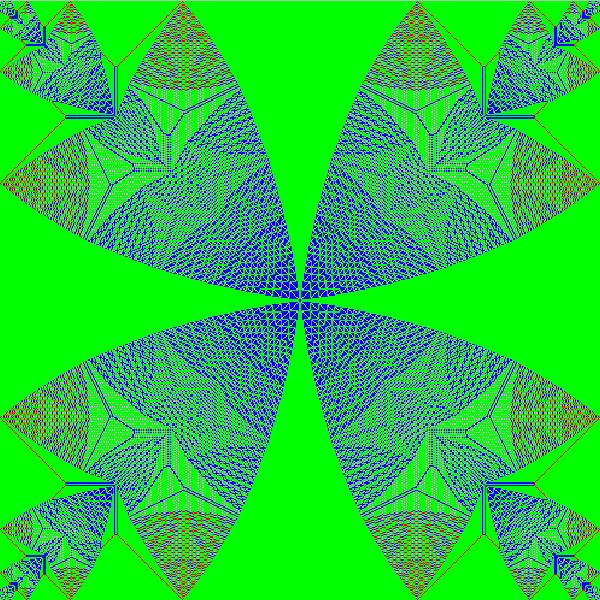Fakultäten der RUB » Fakultät für Mathematik » Lehrstühle » Stochastik und Didaktik

## Shifting Sands

(Jim Propp, University of Wisconsin propp@math.wisc.edu)

Imagine you put 4 grains of sand on each square in a 498-by-498 grid and then update the grid using the following rule: When a square contains four or more grains, it sends one grain to each of the four neighboring squares at the next time-step. (If there are k<4 neighboring squares, as happens at the boundary, the square sends 1 grain to each of its k neighbors, and 4-k of its grains are discarded.) These updates happen simultaneously at all the squares.

When the process is repeated, the system eventually stabilizes, resulting in the picture shown below. Here green, blue, red, and gray pixels denote squares that have 3, 2, 1, and 0 grains, respectively.

The picture was generated using Michael Creutz's program "xsand"; the rule it implements was devised by
physicists Bak, Tang, and Wiesenfeld. Other rules like this are being studied in the Spatial Systems
Laboratory at the University of Wisconsin (http://www.math.wisc.edu/~propp/SSL/